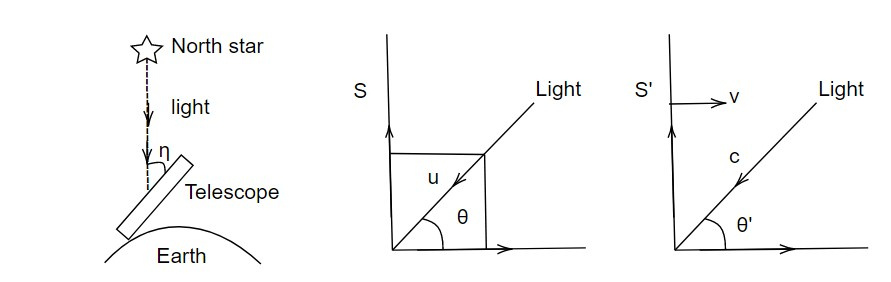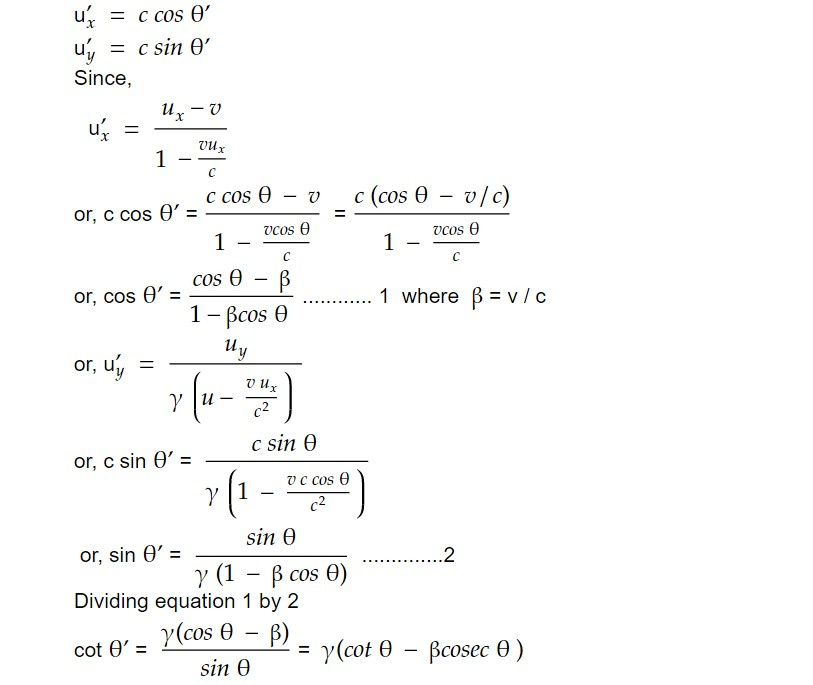# Optical aberration

In astrophysics, optical aberration refers to the distortion or deviation of light as it passes through the Earth's atmosphere or other optical systems, such as telescopes. Optical aberration can cause objects to appear blurry or distorted, and can affect the precision and accuracy of astronomical measurements and observations.

If we like to observe North star, we have to tilt the telescope by a small angle with respect to vertical, this is called optical aberration. To find angular aberration, let light beam be travelling in the direction making an angle θ with X axis in S frame and θ' with X' axis in S' frame component of velocity of light in S frame.ux = c cos θ

uy = c sin θ

in S' frame,The angle of aberration is given by

η = θ' - θ

or, θ' = η + θ

or, cot ( η + θ) = γ (cot θ - βcosecθ )

For North star or Zenith star, β = π/2

So, cot ( η + π/2 ) = γ ( 0 - β )

or, - tan η = - γβ

or, tan η = γβ

For γ -> 1, v<<c

tan η = β

This result is derived by Bardley's Result.